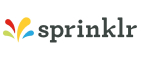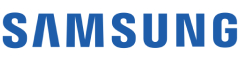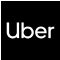New update is available. Click here to update.
Topics

# Return Subsets Sum to K

Moderate0/80
Average time to solve is 40m+5 more companies

## Problem statement

Given an integer array 'ARR' of size 'N' and an integer 'K', return all the subsets of 'ARR' which sum to 'K'.

Subset of an array 'ARR' is a tuple that can be obtained from 'ARR' by removing some (possibly all) elements of 'ARR'.

Note :
``````The order of subsets is not important.

The order of elements in a particular subset should be in increasing order of the index.
``````
Detailed explanation ( Input/output format, Notes, Images )
Constraints:
``````1 <= 'N' <= 16
- (10 ^ 6) <= ARR[i] <= (10 ^ 6)
- 16 * (10 ^ 6) <= 'K' <= 16 * (10 ^ 6)

Where ‘ARR[i]’ denotes the value for ‘ith’ element of the array ‘ARR’ and 'K' is the given sum.

Time Limit: 1 sec.
``````
Sample Input 1:
``````3
2 4 6
6
``````
Sample Output 1:
``````2 4
6
``````
Explanation of the Sample Input 1:
``````For the array'ARR' = {2, 4, 6}, we can have subsets {}, {2}, {4}, {6}, {2, 4}, {2, 6}, {4, 6}, {2, 4, 6}. Out of these 8 subsets, {2, 4} and {6} sum to the given 'K' i.e. 6.
``````
Sample Input 2:
``````6
5 -1 8 2 7 0
7
``````
Sample Output 2:
``````-1 8
-1 8 0
5 2
5 2 0
7
7 0
``````Console